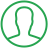# Get Index Of Element In List Python

Last Updated : Jul 1, 2023In this article we will show you the solution of get index of element in list python, Python is a zero-indexed language, which means that elements are labeled from 0 and not 1.

Indexing is important while working with strings, lists, dictionaries, and other data structures in Python.

Here, we are going to find the index of a specific element in a list in Python.

## Step By Step Guide On Get Index Of Element In List Python :-

There are several ways to find the index of a specific element in a list in Python.

One way to do this is by iterating over the list and then, initializing the counter variable starting from zero and increasing the counter variable by one as we go through each element of the iteration.

And when we reach the specific element whose index we were looking for, we will print the counter variable, and break the loop.

This will give us the index of the specific element.

But this is a complex method and also it requires a large time and space complexity as we are iterating over each element and also comparing the elements to know if it's the right element.

In order to deal with this we will use the built-in index() function, which is a lot simpler.

index() function:

`Syntax: list.index(element)`

Use: index function gives the index of the element specified. If there are many occurrences of the element then only the index of the first element is given.

So if the element doesn't exist in the list then we get an 'item is not in list' error.

```students = ["Alby", "Newt", "Thomas", "Frypan", "Brenda", "Newt", "Minho", "Gally"]
index = students.index("Brenda");
print(f"The index of the element \"Brenda\" is {index}."));
index = students.index("Newt");
print(f"The index of the element \"Newt\" is {index}.");
index = students.index("John");
print(f"The index of the element \"John\" is {index}");

#Code Output
The index of the element "Brenda" is 4.
The index of the element "Newt" is 1.
ValueError: 'John' is not in list```
1. First, initialize a list named students with the name of students in it.
2. After that, we have taken a variable named index and used the built-in index function, and passed the element of which we have to find the index. Also, in the place of the list, we have placed the name of the list which is students.
3. After that, we printed the index given by the built-in index function.
4. As we can see in the output the index of the element print is 4.
5. For the element, Newt the index will be 1 but we can see that they are more than two occurrences of the same element.
6. As mentioned in the use cases the first occurrence of the element is considered so the index of new will be 1.
7. So in the next example, the element specified is John.
8. We can clearly see the element John is not present in our list so when we try to print the statement we get the value error John is not in list.

## Conclusion :-

This is how we get the index of the element in the list in Python. We have already seen various test cases of the index built-in function. Give it a try and find more such cases.

I hope this article on get index of element in list python helps you and the steps and method mentioned above are easy to follow and implement.

##About Anjali

Experienced Computer Programmer with a broad range of experience in technology. Strengths in application development and Object Oriented architecture design, front end programming, usability and multimedia technology. Expert in coding languages such as C, C++ Java, JavaScript, PHP and more.